Скачать презентацию Lecture Slides for INTRODUCTION TO Machine Learning 2

770a43896307f05bfdbb93d89dc47e4c.ppt

• Количество слайдов: 30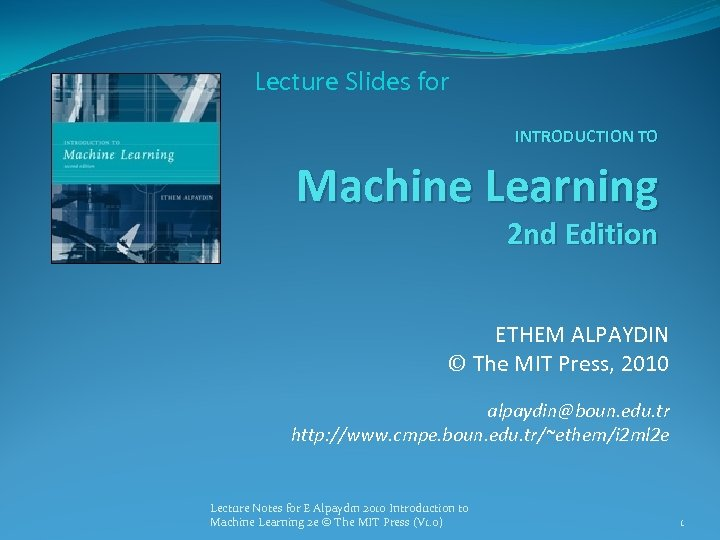Lecture Slides for INTRODUCTION TO Machine Learning 2 nd Edition ETHEM ALPAYDIN © The MIT Press, 2010 [email protected] edu. tr http: //www. cmpe. boun. edu. tr/~ethem/i 2 ml 2 e Lecture Notes for E Alpaydın 2010 Introduction to Machine Learning 2 e © The MIT Press (V 1. 0) 1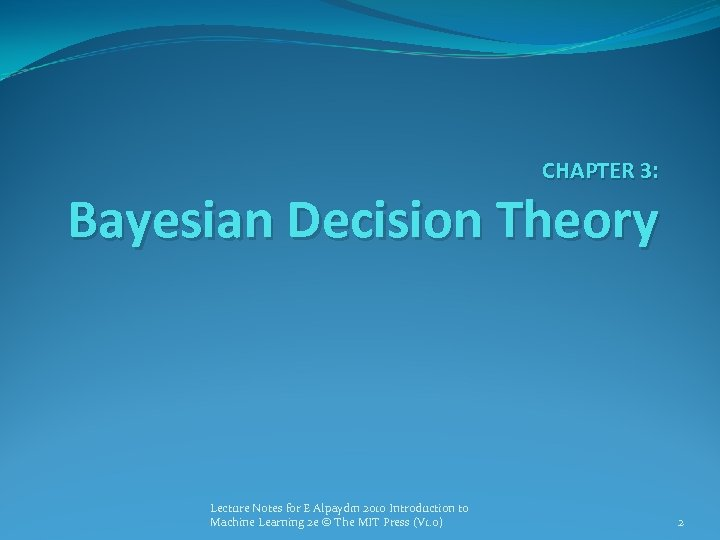CHAPTER 3: Bayesian Decision Theory Lecture Notes for E Alpaydın 2010 Introduction to Machine Learning 2 e © The MIT Press (V 1. 0) 2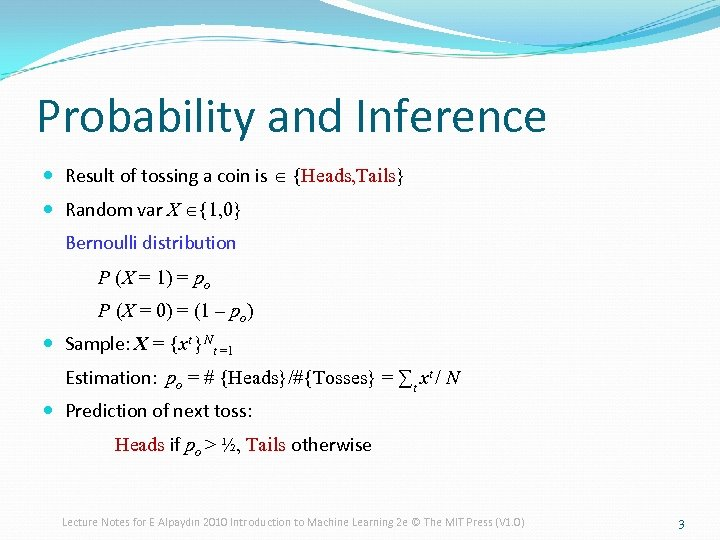Probability and Inference Result of tossing a coin is Î {Heads, Tails} Random var X Î{1, 0} Bernoulli distribution P (X = 1) = po P (X = 0) = (1 ‒ po) Sample: X = {xt }Nt =1 Estimation: po = # {Heads}/#{Tosses} = ∑t xt / N Prediction of next toss: Heads if po > ½, Tails otherwise Lecture Notes for E Alpaydın 2010 Introduction to Machine Learning 2 e © The MIT Press (V 1. 0) 3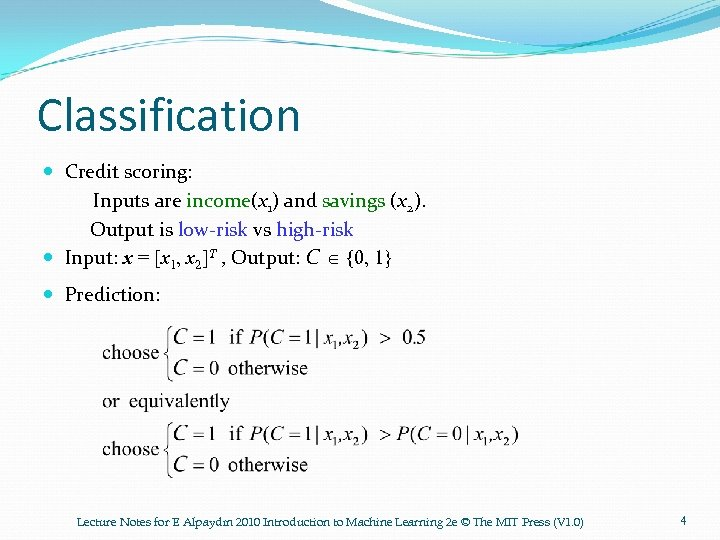Classification Credit scoring: Inputs are income(x 1) and savings (x 2). Output is low-risk vs high-risk Input: x = [x 1, x 2]T , Output: C Î {0, 1} Prediction: Lecture Notes for E Alpaydın 2010 Introduction to Machine Learning 2 e © The MIT Press (V 1. 0) 4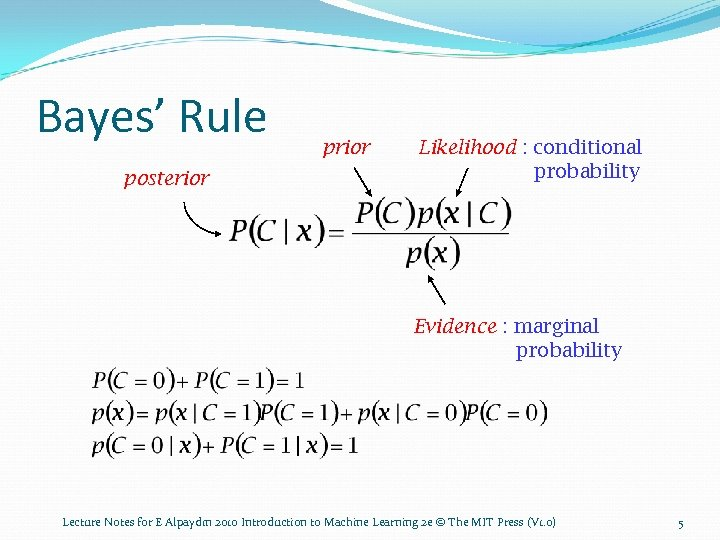Bayes’ Rule posterior prior Likelihood : conditional probability Evidence : marginal probability Lecture Notes for E Alpaydın 2010 Introduction to Machine Learning 2 e © The MIT Press (V 1. 0) 5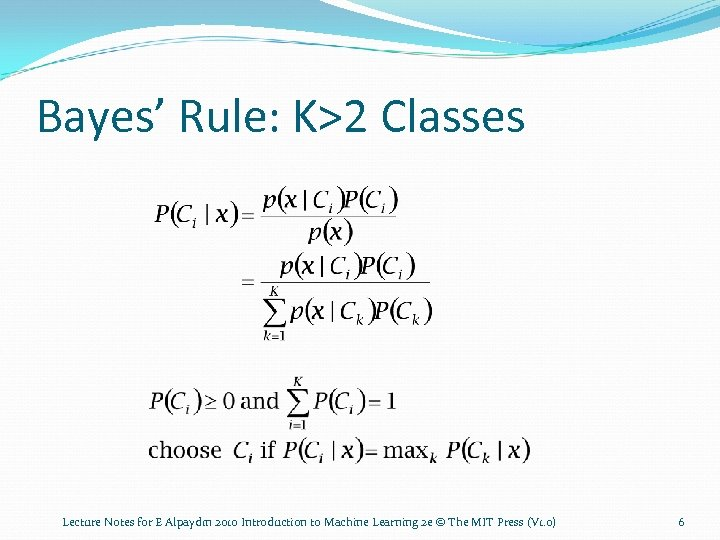Bayes’ Rule: K>2 Classes Lecture Notes for E Alpaydın 2010 Introduction to Machine Learning 2 e © The MIT Press (V 1. 0) 6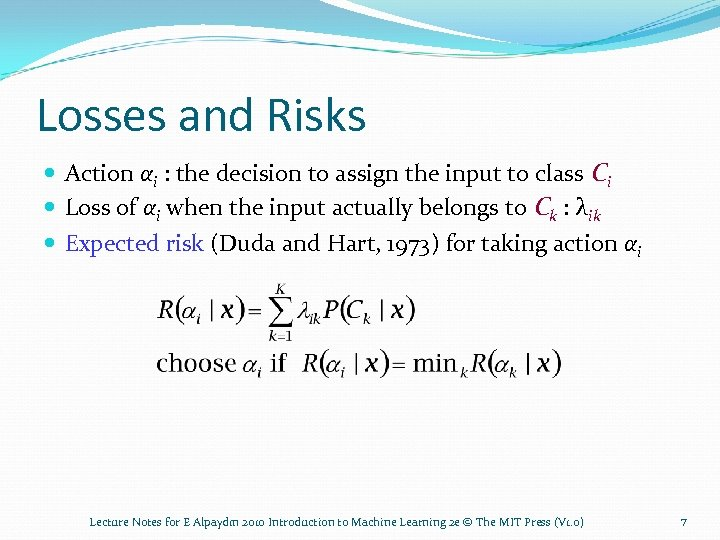Losses and Risks Action αi : the decision to assign the input to class Ci Loss of αi when the input actually belongs to Ck : λik Expected risk (Duda and Hart, 1973) for taking action αi Lecture Notes for E Alpaydın 2010 Introduction to Machine Learning 2 e © The MIT Press (V 1. 0) 7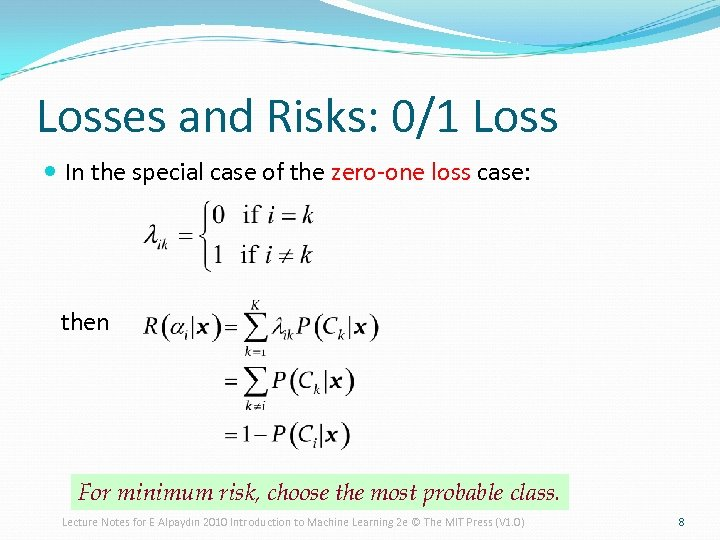Losses and Risks: 0/1 Loss In the special case of the zero-one loss case: then For minimum risk, choose the most probable class. Lecture Notes for E Alpaydın 2010 Introduction to Machine Learning 2 e © The MIT Press (V 1. 0) 8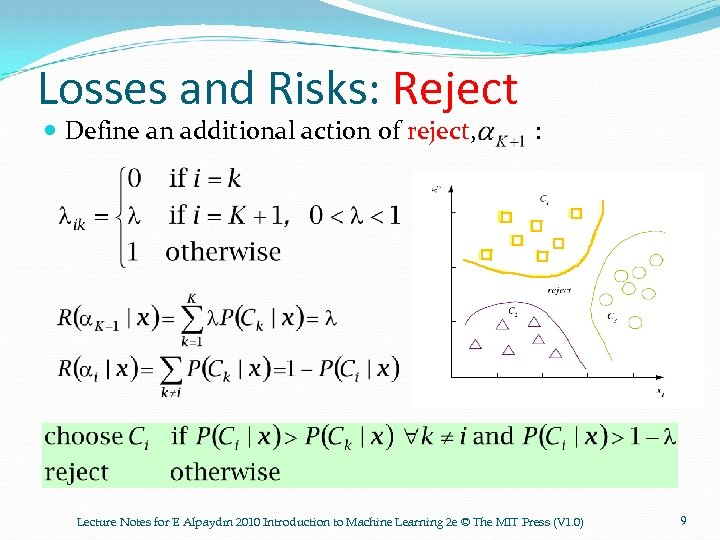Losses and Risks: Reject Define an additional action of reject, : Lecture Notes for E Alpaydın 2010 Introduction to Machine Learning 2 e © The MIT Press (V 1. 0) 9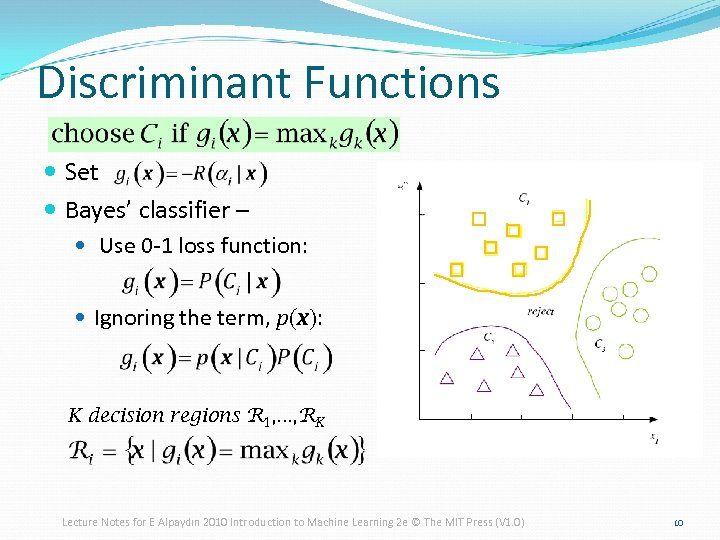Discriminant Functions Set Bayes’ classifier – Use 0 -1 loss function: Ignoring the term, p(x): K decision regions R 1, . . . , RK Lecture Notes for E Alpaydın 2010 Introduction to Machine Learning 2 e © The MIT Press (V 1. 0) 10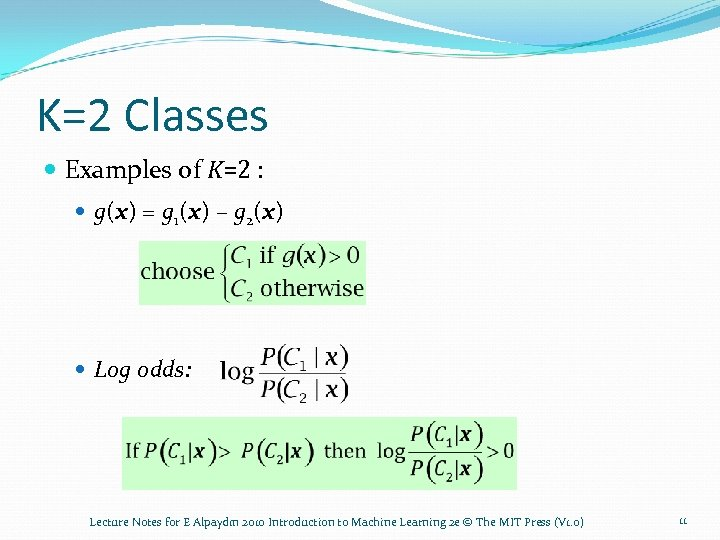K=2 Classes Examples of K=2 : g(x) = g 1(x) – g 2(x) Log odds: Lecture Notes for E Alpaydın 2010 Introduction to Machine Learning 2 e © The MIT Press (V 1. 0) 11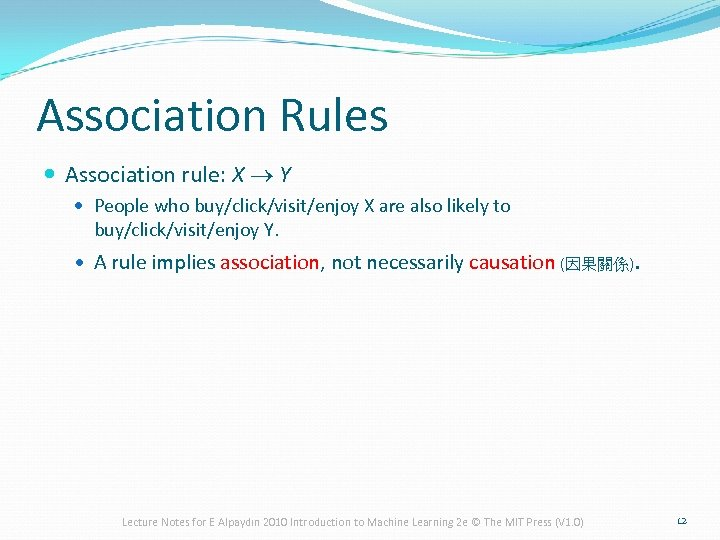Association Rules Association rule: X ® Y People who buy/click/visit/enjoy X are also likely to buy/click/visit/enjoy Y. A rule implies association, not necessarily causation (因果關係). Lecture Notes for E Alpaydın 2010 Introduction to Machine Learning 2 e © The MIT Press (V 1. 0) 12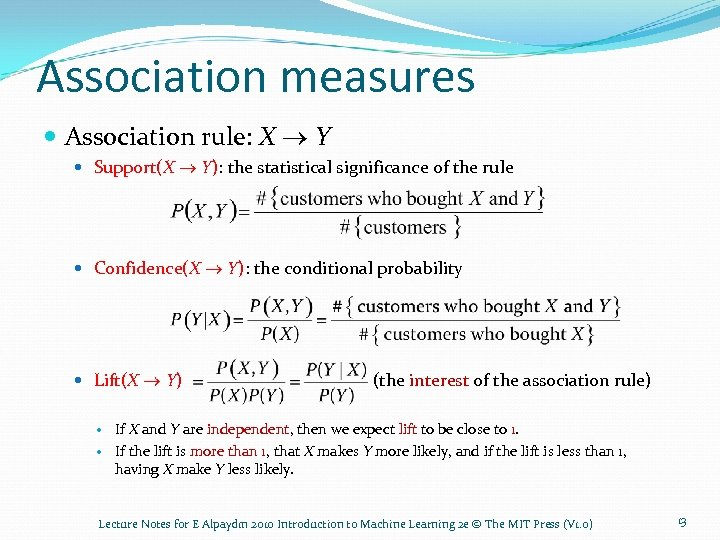Association measures Association rule: X ® Y Support(X ® Y): the statistical significance of the rule Confidence(X ® Y): the conditional probability Lift(X ® Y) (the interest of the association rule) If X and Y are independent, then we expect lift to be close to 1. If the lift is more than 1, that X makes Y more likely, and if the lift is less than 1, having X make Y less likely. Lecture Notes for E Alpaydın 2010 Introduction to Machine Learning 2 e © The MIT Press (V 1. 0) 13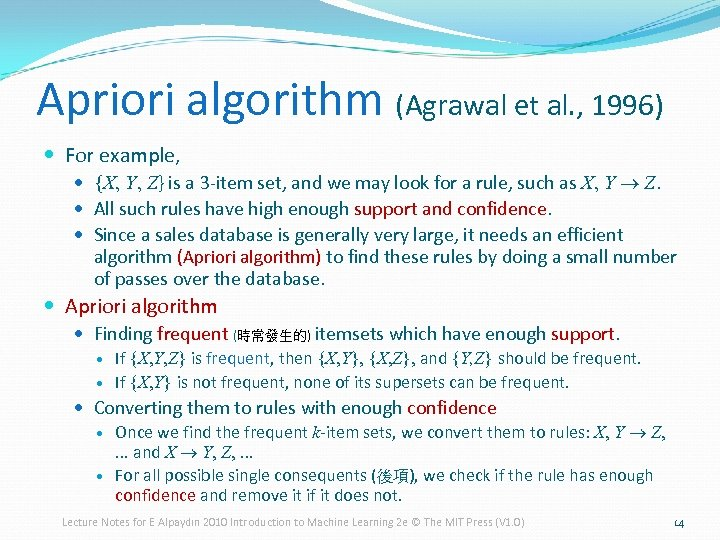Apriori algorithm (Agrawal et al. , 1996) For example, {X, Y, Z} is a 3 -item set, and we may look for a rule, such as X, Y ® Z. All such rules have high enough support and confidence. Since a sales database is generally very large, it needs an efficient algorithm (Apriori algorithm) to find these rules by doing a small number of passes over the database. Apriori algorithm Finding frequent (時常發生的) itemsets which have enough support. If {X, Y, Z} is frequent, then {X, Y}, {X, Z}, and {Y, Z} should be frequent. If {X, Y} is not frequent, none of its supersets can be frequent. Converting them to rules with enough confidence Once we find the frequent k-item sets, we convert them to rules: X, Y ® Z, . . . and X ® Y, Z, . . . For all possible single consequents (後項), we check if the rule has enough confidence and remove it if it does not. Lecture Notes for E Alpaydın 2010 Introduction to Machine Learning 2 e © The MIT Press (V 1. 0) 14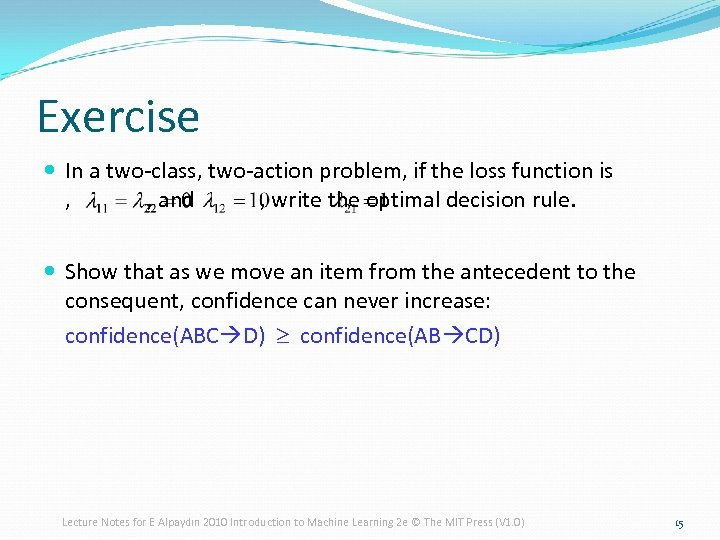Exercise In a two-class, two-action problem, if the loss function is , , and , write the optimal decision rule. Show that as we move an item from the antecedent to the consequent, confidence can never increase: confidence(ABC D) confidence(AB CD) Lecture Notes for E Alpaydın 2010 Introduction to Machine Learning 2 e © The MIT Press (V 1. 0) 15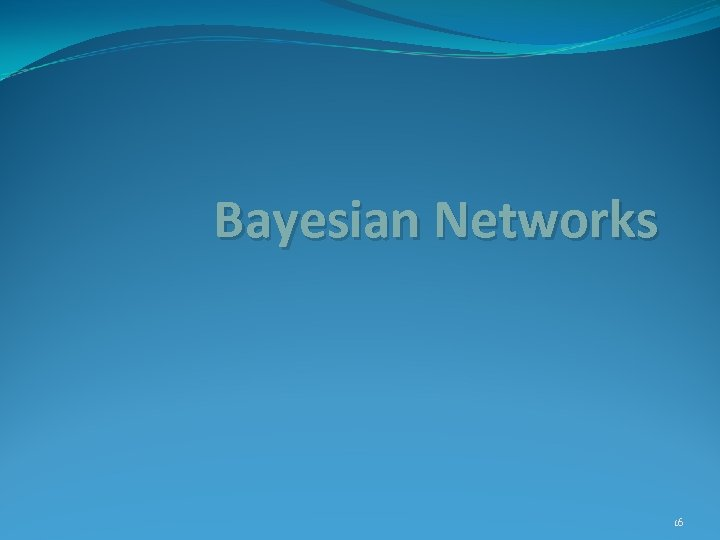Bayesian Networks 16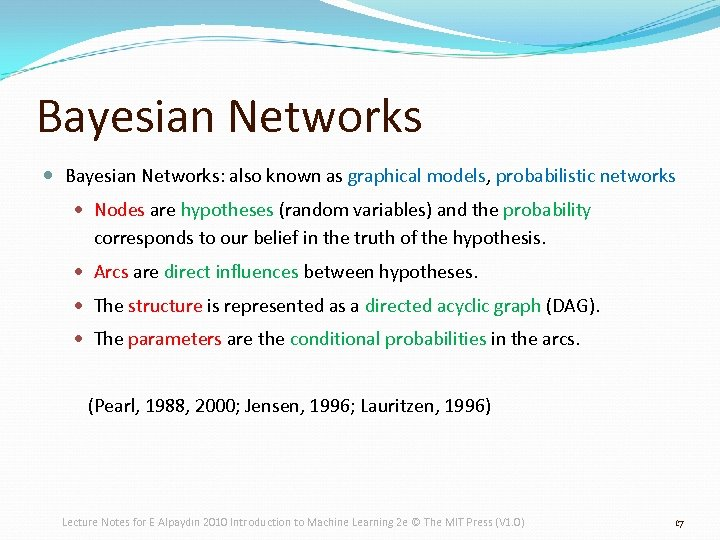Bayesian Networks Bayesian Networks: also known as graphical models, probabilistic networks Nodes are hypotheses (random variables) and the probability corresponds to our belief in the truth of the hypothesis. Arcs are direct influences between hypotheses. The structure is represented as a directed acyclic graph (DAG). The parameters are the conditional probabilities in the arcs. (Pearl, 1988, 2000; Jensen, 1996; Lauritzen, 1996) Lecture Notes for E Alpaydın 2010 Introduction to Machine Learning 2 e © The MIT Press (V 1. 0) 17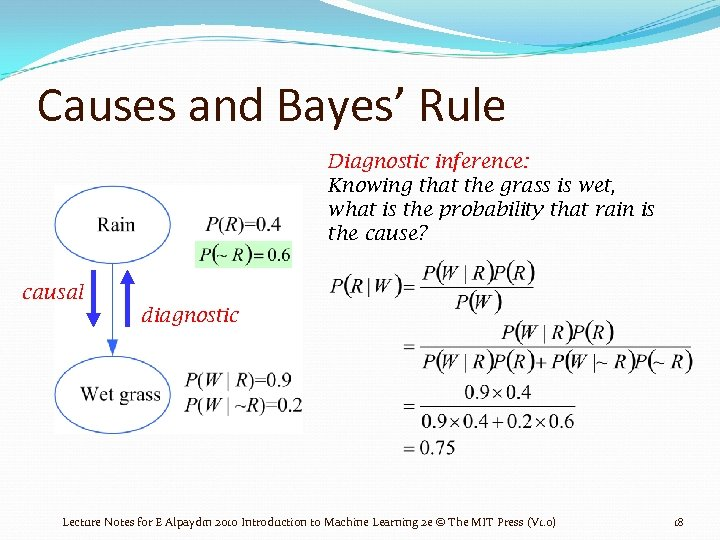Causes and Bayes’ Rule Diagnostic inference: Knowing that the grass is wet, what is the probability that rain is the cause? causal diagnostic Lecture Notes for E Alpaydın 2010 Introduction to Machine Learning 2 e © The MIT Press (V 1. 0) 18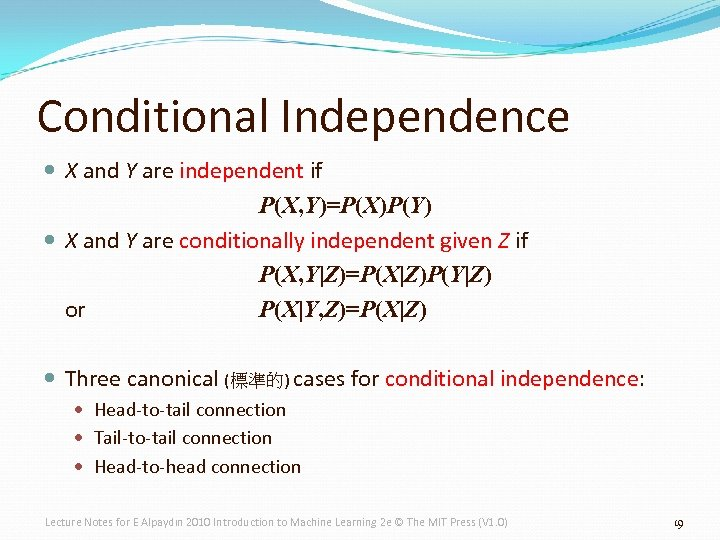Conditional Independence X and Y are independent if P(X, Y)=P(X)P(Y) X and Y are conditionally independent given Z if P(X, Y|Z)=P(X|Z)P(Y|Z) or P(X|Y, Z)=P(X|Z) Three canonical (標準的) cases for conditional independence: Head-to-tail connection Tail-to-tail connection Head-to-head connection Lecture Notes for E Alpaydın 2010 Introduction to Machine Learning 2 e © The MIT Press (V 1. 0) 19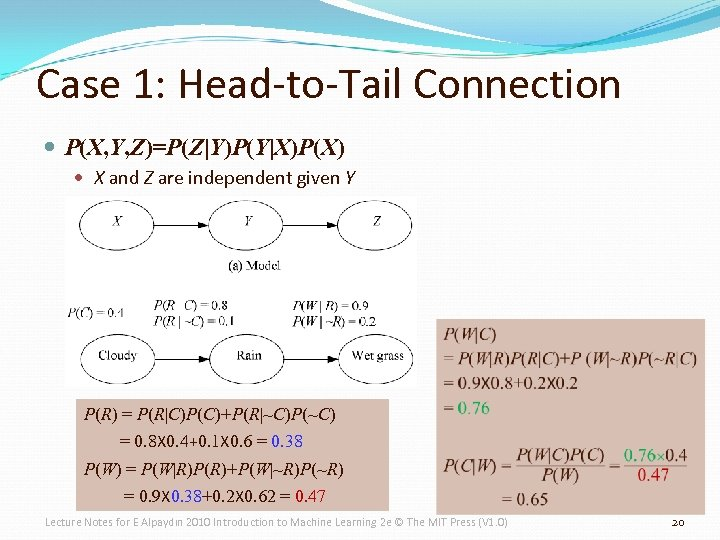Case 1: Head-to-Tail Connection P(X, Y, Z)=P(Z|Y)P(Y|X)P(X) X and Z are independent given Y P(R) = P(R|C)P(C)+P(R|~C)P(~C) = 0. 8 X 0. 4+0. 1 X 0. 6 = 0. 38 P(W) = P(W|R)P(R)+P(W|~R)P(~R) = 0. 9 X 0. 38+0. 2 X 0. 62 = 0. 47 Lecture Notes for E Alpaydın 2010 Introduction to Machine Learning 2 e © The MIT Press (V 1. 0) 20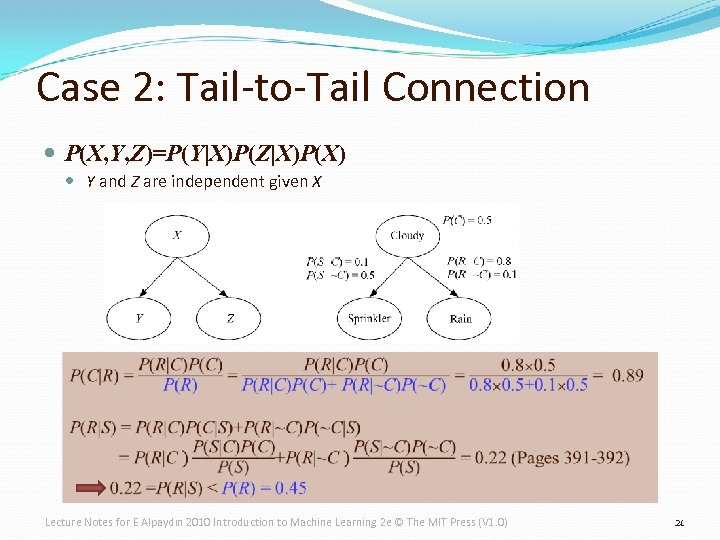Case 2: Tail-to-Tail Connection P(X, Y, Z)=P(Y|X)P(Z|X)P(X) Y and Z are independent given X Lecture Notes for E Alpaydın 2010 Introduction to Machine Learning 2 e © The MIT Press (V 1. 0) 21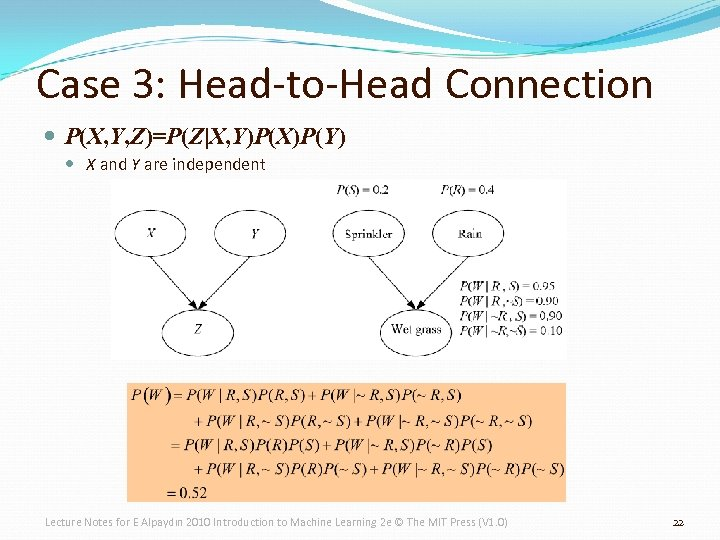Case 3: Head-to-Head Connection P(X, Y, Z)=P(Z|X, Y)P(X)P(Y) X and Y are independent Lecture Notes for E Alpaydın 2010 Introduction to Machine Learning 2 e © The MIT Press (V 1. 0) 22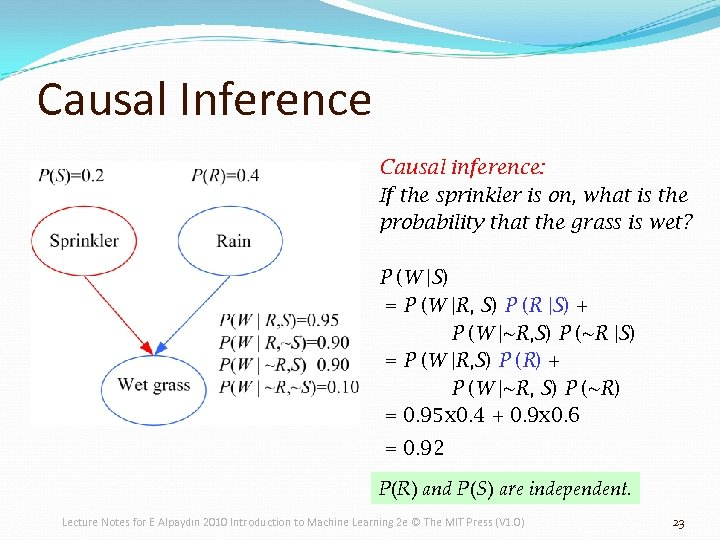Causal Inference Causal inference: If the sprinkler is on, what is the probability that the grass is wet? P (W |S) = P (W |R, S) P (R |S) + P (W |~R, S) P (~R |S) = P (W |R, S) P (R) + P (W |~R, S) P (~R) = 0. 95 x 0. 4 + 0. 9 x 0. 6 = 0. 92 P(R) and P(S) are independent. Lecture Notes for E Alpaydın 2010 Introduction to Machine Learning 2 e © The MIT Press (V 1. 0) 23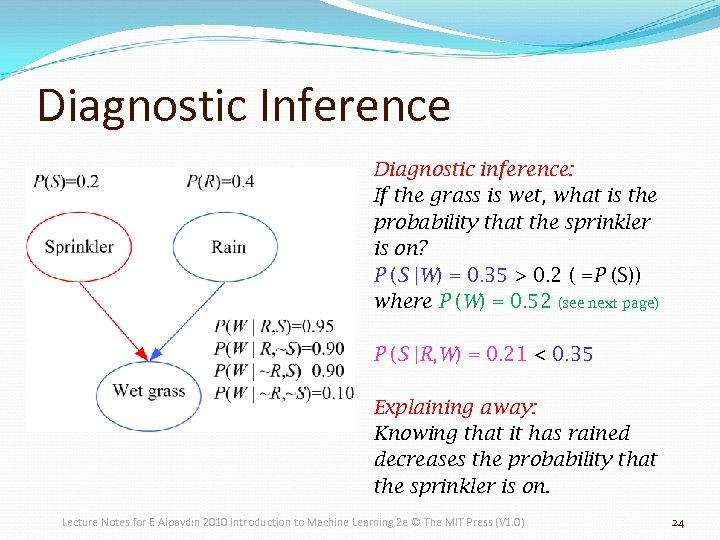Diagnostic Inference Diagnostic inference: If the grass is wet, what is the probability that the sprinkler is on? P (S |W) = 0. 35 > 0. 2 ( =P (S)) where P (W) = 0. 52 (see next page) P (S |R, W) = 0. 21 < 0. 35 Explaining away: Knowing that it has rained decreases the probability that the sprinkler is on. Lecture Notes for E Alpaydın 2010 Introduction to Machine Learning 2 e © The MIT Press (V 1. 0) 24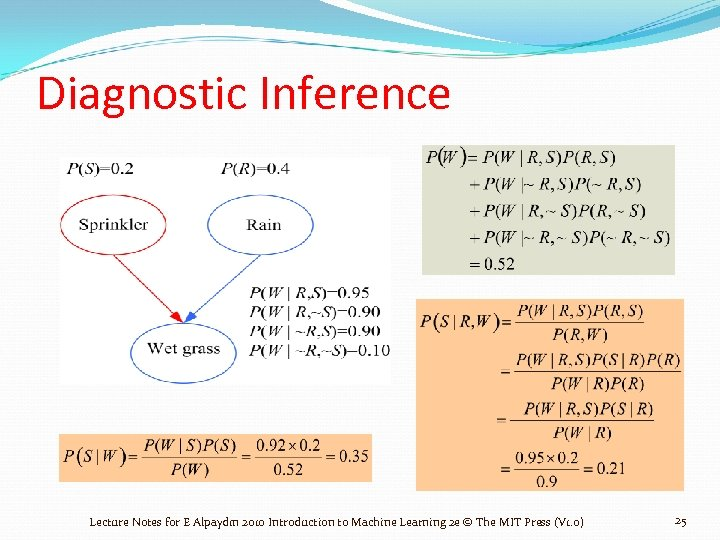Diagnostic Inference Lecture Notes for E Alpaydın 2010 Introduction to Machine Learning 2 e © The MIT Press (V 1. 0) 25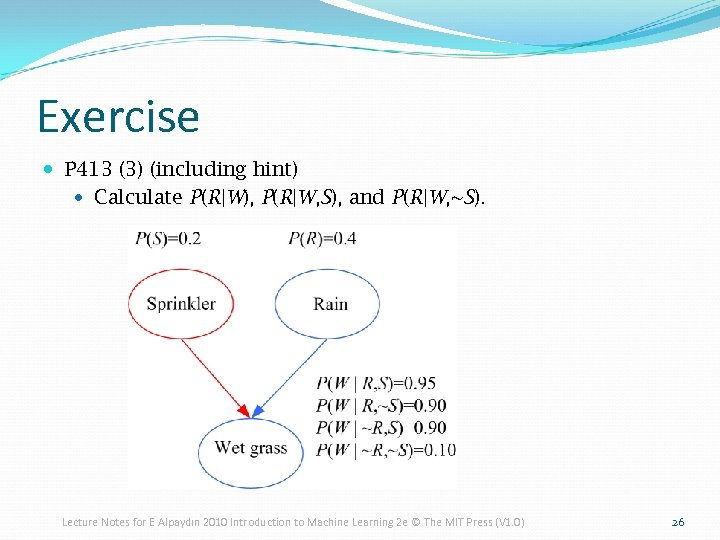Exercise P 413 (3) (including hint) Calculate P(R|W), P(R|W, S), and P(R|W, ~S). Lecture Notes for E Alpaydın 2010 Introduction to Machine Learning 2 e © The MIT Press (V 1. 0) 26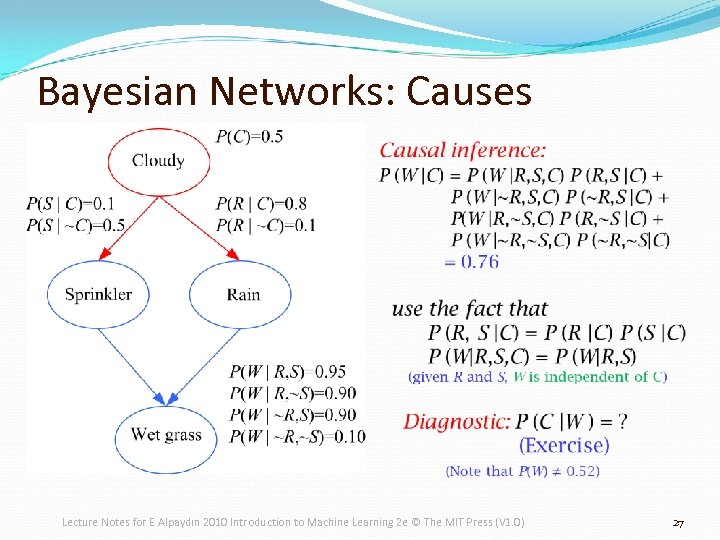Bayesian Networks: Causes Lecture Notes for E Alpaydın 2010 Introduction to Machine Learning 2 e © The MIT Press (V 1. 0) 27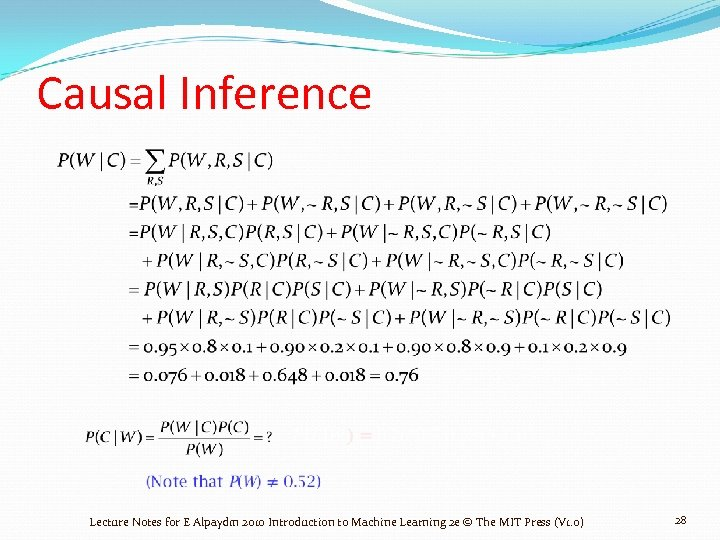Causal Inference Lecture Notes for E Alpaydın 2010 Introduction to Machine Learning 2 e © The MIT Press (V 1. 0) 28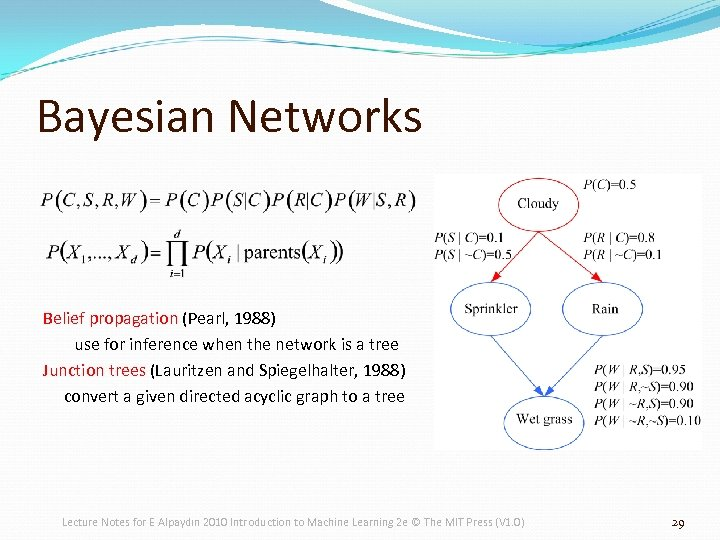Bayesian Networks Belief propagation (Pearl, 1988) use for inference when the network is a tree Junction trees (Lauritzen and Spiegelhalter, 1988) convert a given directed acyclic graph to a tree Lecture Notes for E Alpaydın 2010 Introduction to Machine Learning 2 e © The MIT Press (V 1. 0) 29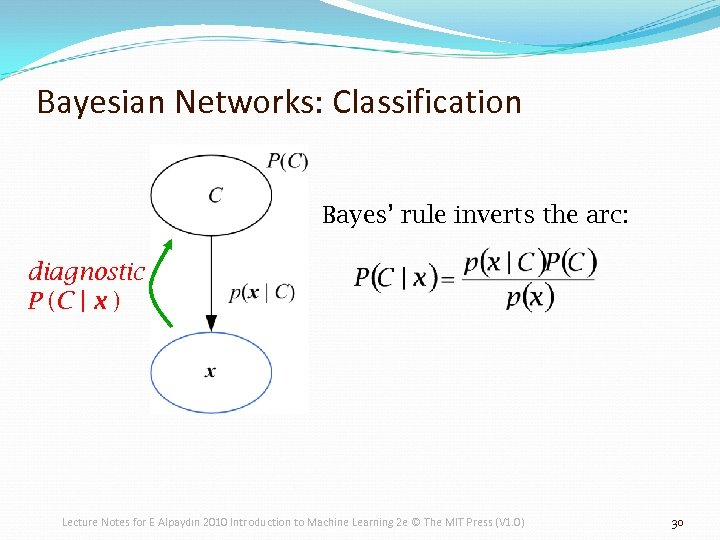Bayesian Networks: Classification Bayes’ rule inverts the arc: diagnostic P (C | x ) Lecture Notes for E Alpaydın 2010 Introduction to Machine Learning 2 e © The MIT Press (V 1. 0) 30